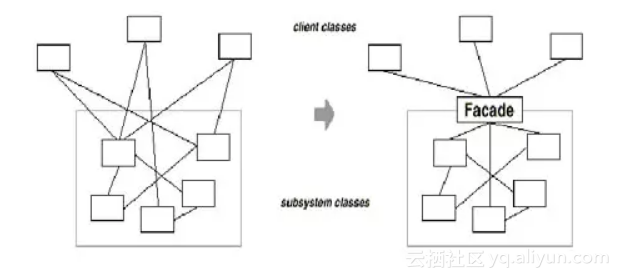#1. 云栖社区>
2. Java杂记>
3. 博客>
4. 正文

1. `public class DBCompare {`

2. ` Connection conn = null;`

3. ` PreparedStatement prep = null;`

4. ` ResultSet rset = null;`

5. ` try {`

6. ` Class.forName( "<driver>" ).newInstance();`

7. `　　conn = DriverManager.getConnection( "<database>" );`

8. ` `
9. ` String sql = "SELECT * FROM <table> WHERE <column name> = ?";`

10. `　　prep = conn.prepareStatement( sql );`

11. `　 prep.setString( 1, "<column value>" );`

12. `　　rset = prep.executeQuery();`

13. ` if( rset.next() ) {`

14. ` System.out.println( rset.getString( "<column name" ) );`

15. ` }`

16. ` } catch( SException e ) {`

17. `　　e.printStackTrace();`

18. ` } finally {`

19. `　　rset.close();`

20. `　　prep.close();`

21. `　　conn.close();`

22. ` }`

23. `}`

1. `public class DBCompare {`

2. ` String sql = "SELECT * FROM <table> WHERE <column name> = ?";　　`

3. ` try {`

4. ` Mysql msql=new mysql(sql);`

5. `　　prep.setString( 1, "<column value>" );`

6. `　　rset = prep.executeQuery();`

7. ` if( rset.next() ) {`

8. ` System.out.println( rset.getString( "<column name" ) );`

9. ` }`

10. ` } catch( SException e ) {`

11. `　　e.printStackTrace();`

12. ` } finally {`

13. `　　mysql.close();`

14. `　　mysql=null;`

15. ` }`

16. `}`